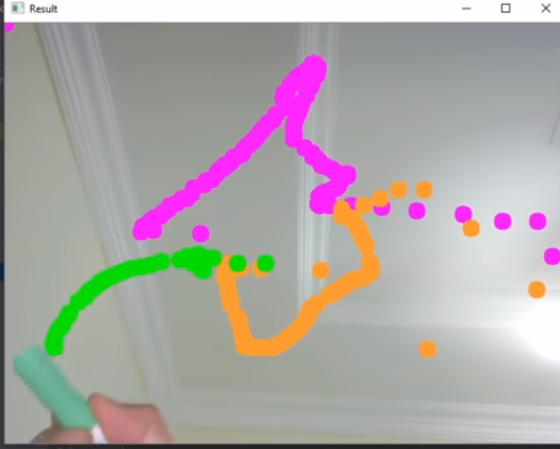# Live Webcam Drawing using OpenCV

• Difficulty Level : Expert
• Last Updated : 22 Feb, 2022

Let us see how to draw the movement of objects captured by the webcam using OpenCV. Our program takes the video input from the webcam and tracks the objects we are moving. After identifying the objects, it will make contours precisely. After that, it will print all your drawing on the output screen.

## python3

 `# importing the modules``import` `cv2``import` `numpy as np`` ` `# set Width and Height of output Screen``frameWidth ``=` `640``frameHeight ``=` `480`` ` `# capturing Video from Webcam``cap ``=` `cv2.VideoCapture(``0``)``cap.``set``(``3``, frameWidth)``cap.``set``(``4``, frameHeight)`` ` `# set brightness, id is 10 and``# value can be changed accordingly``cap.``set``(``10``,``150``)``  ` `# object color values``myColors ``=` `[[``5``, ``107``, ``0``, ``19``, ``255``, ``255``],``            ``[``133``, ``56``, ``0``, ``159``, ``156``, ``255``],``            ``[``57``, ``76``, ``0``, ``100``, ``255``, ``255``],``            ``[``90``, ``48``, ``0``, ``118``, ``255``, ``255``]]`` ` `# color values which will be used to paint``# values needs to be in BGR``myColorValues ``=` `[[``51``, ``153``, ``255``],         ``                 ``[``255``, ``0``, ``255``],``                 ``[``0``, ``255``, ``0``],          ``                 ``[``255``, ``0``, ``0``]]` `# [x , y , colorId ]``myPoints ``=` `[] ``   ` `# function to pick color of object``def` `findColor(img, myColors, myColorValues):` `    ``# converting the image to HSV format``    ``imgHSV ``=` `cv2.cvtColor(img, cv2.COLOR_BGR2HSV)``    ``count ``=` `0``    ``newPoints ``=` `[]``     ` `    ``# running for loop to work with all colors``    ``for` `color ``in` `myColors:``        ``lower ``=` `np.array(color[``0``:``3``])``        ``upper ``=` `np.array(color[``3``:``6``])``        ``mask ``=` `cv2.inRange(imgHSV,lower,upper)``        ``x, y ``=` `getContours(mask)` `        ``# making the circles``        ``cv2.circle(imgResult, (x,y), ``15``,``                   ``myColorValues[count], cv2.FILLED)``        ``if` `x !``=` `0` `and` `y !``=` `0``:``            ``newPoints.append([x,y,count])``        ``count ``+``=` `1``    ``return` `newPoints``  ` ` ` `# contours function used to improve accuracy of paint``def` `getContours(img):``    ``_, contours, hierarchy ``=` `cv2.findContours(img, cv2.RETR_EXTERNAL,``                                              ``cv2.CHAIN_APPROX_NONE)``    ``x, y, w, h ``=` `0``, ``0``, ``0``, ``0``     ` `    ``# working with contours``    ``for` `cnt ``in` `contours:``        ``area ``=` `cv2.contourArea(cnt)``        ``if` `area > ``500``:``            ``peri ``=` `cv2.arcLength(cnt, ``True``)``            ``approx ``=` `cv2.approxPolyDP(cnt, ``0.02` `*` `peri, ``True``)``            ``x, y, w, h ``=` `cv2.boundingRect(approx)``    ``return` `x ``+` `w ``/``/` `2``, y``  ` ` ` `# draws your action on virtual canvas``def` `drawOnCanvas(myPoints, myColorValues):``    ``for` `point ``in` `myPoints:``        ``cv2.circle(imgResult, (point[``0``], point[``1``]),``                   ``10``, myColorValues[point[``2``]], cv2.FILLED)``  ` `# running infinite while loop so that``# program keep running until we close it``while` `True``:``    ``success, img ``=` `cap.read()``    ``imgResult ``=` `img.copy()` `    ``# finding the colors for the points``    ``newPoints ``=` `findColor(img, myColors, myColorValues)``    ``if` `len``(newPoints)!``=` `0``:``        ``for` `newP ``in` `newPoints:``            ``myPoints.append(newP)``    ``if` `len``(myPoints)!``=` `0``:` `        ``# drawing the points``        ``drawOnCanvas(myPoints, myColorValues)``  ` `    ``# displaying output on Screen``    ``cv2.imshow("Result", imgResult)``     ` `    ``# condition to break programs execution``    ``# press q to stop the execution of program``    ``if` `cv2.waitKey(``1``) ``and` `0xFF` `=``=` `ord``(``'q'``):``        ``break`

Output :My Personal Notes arrow_drop_up